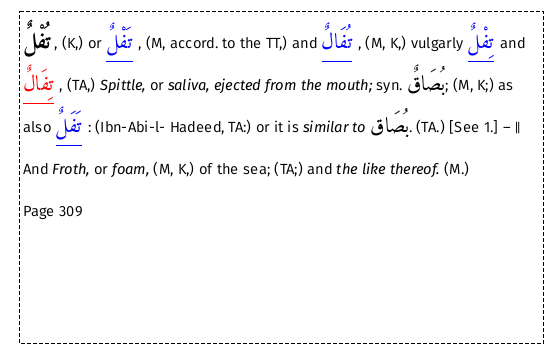This section contains a brief overview of the XML structure of a Lexicon entry.

For users familiar with XSLT, the distributed stylesheet contains comments that may be of assistance.

The XML structure of a Lexicon entry is illustrated using the following example:To view this entry search for node 3972.

``````<word buck="tufolN" ar="تُفْلٌ" page="309" itype="" supp="0">
<entryFree id="n3972" key="تُفْلٌ" type="main">
<form>
<orth orig="" extent="full" lang="ar">تُفْلٌ</orth>
<orth extent="full" lang="ar">فال</orth>
<orth extent="full" lang="ar">فل</orth>
<orth extent="full" lang="ar">فلى</orth>
<orth extent="full" lang="ar">تفل</orth>
</form>, (K,) or
<foreign lang="ar">
<ref cref="n3972-1" target="تَفْلٌ" n="1" type="1" subtype="WO" lang="ar" select="n3971"/>
</foreign>, (M, accord. to the TT,) and
<foreign lang="ar">
<ref cref="n3972-2" target="تُفَالٌ" n="2" type="1" subtype="WO" lang="ar" select="n3976"/>
</foreign>, (M, K,) vulgarly
<foreign lang="ar">
<ref cref="n3972-3" target="تِفْلٌ" n="3" type="1" subtype="WO" lang="ar" select="n3973"/>
</foreign> and
<foreign lang="ar">
<ref cref="n3972-4" target="تِفَالٌ" n="4" type="1" subtype="WO" lang="ar"/>
</foreign>, (TA,) <hi rend="ital">Spittle,</hi> or <hi rend="ital">saliva, ejected from the mouth;</hi> syn. <foreign lang="ar">بُصَاقٌ</foreign>; (M, K;) as also
<foreign lang="ar">
<ref cref="n3972-5" target="تَفَلٌ" n="5" type="1" subtype="WO" lang="ar" select="n3974"/>
</foreign>: (Ibn-Abi-l- Hadeed, TA:) or it is <hi rend="ital">similar to</hi>
<foreign lang="ar">بُصَاق</foreign>. (TA.) [See 1.] ―
<sense type="b" n="2">-b2-</sense>  And <hi rend="ital">Froth,</hi> or <hi rend="ital">foam,</hi> (M, K,) of the sea; (TA;) and <hi rend="ital">the like thereof.</hi> (M.)
<pb n="309"/>
</entryFree>
</word>

``````

It should be noted that the surrounding <word> tags are not stored in the database but are inserted as the entry is loaded. The XSLT stylesheet expects these tags to be present.

<word>

``````Most of the attributes are informational only whose meaning should be clear.

"supp"  value of 1 indicates the entry is from the supplement

Root entries are identified by

"type"  attribute with a value of "root"
"quasi" is also present for roots described as "Quasi"
``````

<form> ignored

<ref> Cross reference. These are converted to links.

``````the presence of a "select" attribute indicates that the destination of the cross-reference has been
identifed and the  value of the attribute is the target node.
``````

<sense> sense separator

``````The "type" attribute has two values:
a    denotes "an extraordinary, or a complete, dissociation."
b   "to denote a break in the relations of significations"
``````

<pb> Page break.